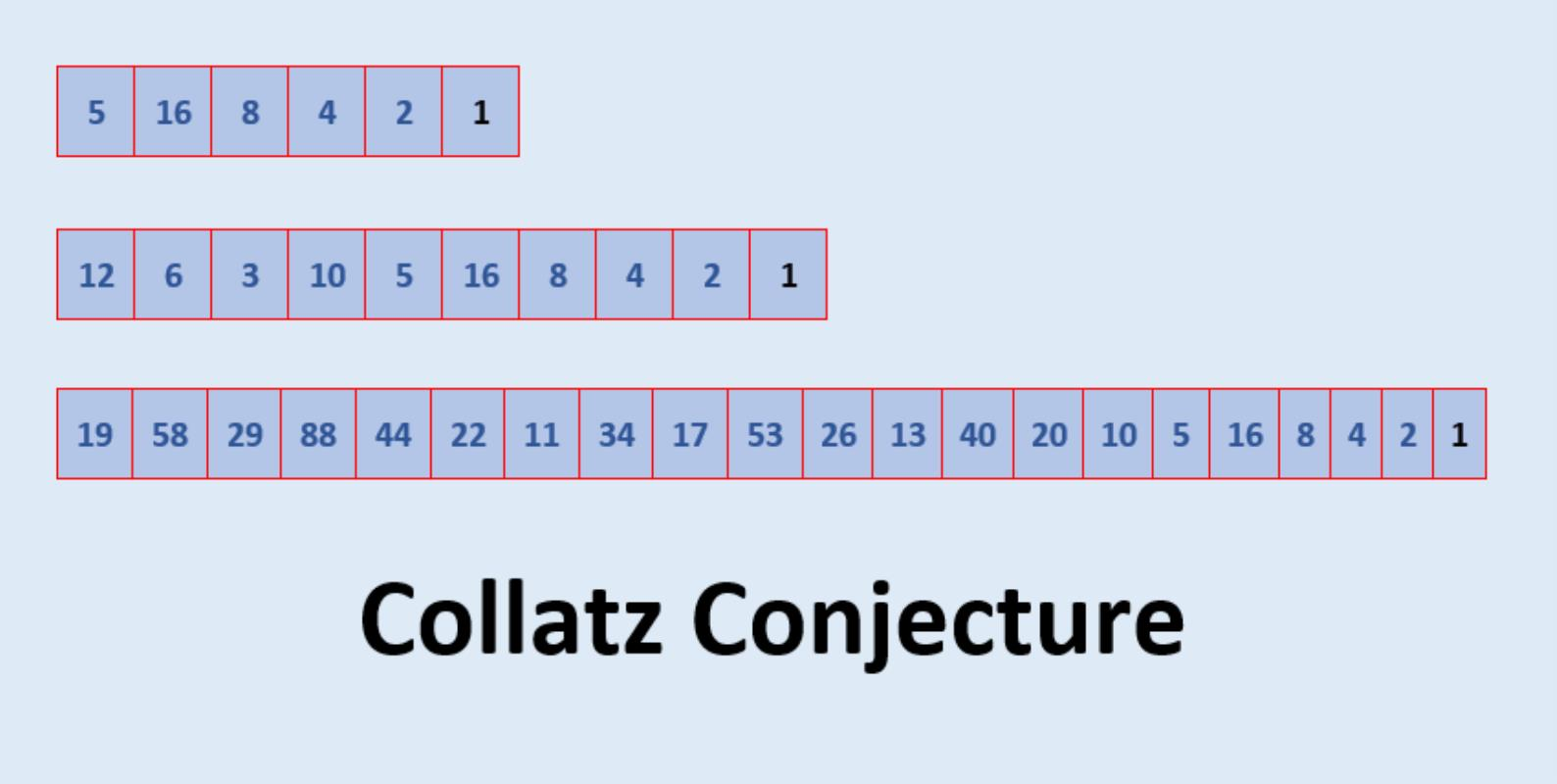# The "simplest" unsolved problem in mathematicsMathematics is filled with unsolved problems. Not surprisingly, most of these problems are quite technical.

To understand them, let alone solve them, one needs a strong mathematics background. Take for example the so-called millennium problems.

This is a list of seven problems that were stated by the Clay Mathematics Institute in the year 2000 each carrying a monetary prize of \$1,000,000 for a correct solution (note that one of these problems, the Poincare conjecture, was solved by Grigori Perelman in 2006 but he declined the monetary prize). These millennium problems are very technical; a thorough understanding of each problem would probably require a PhD in mathematics.

Here I will not be technical at all; I will state what is probably the simplest unsolved problem in mathematics to understand. This problem is known as the Collatz conjecture, the 3x+1 conjecture, the Ulam conjecture and there are many other names for it.

This problem that was proposed in 1937 goes as follows: Choose any positive whole number greater than 1. From this number we create a sequence by applying the following two rules: If the number we have is odd, multiply it by 3 and add 1. If the number is even, divide it by 2. The problem is to show that the sequence we obtain must eventually reach 1.

For example, lets begin with the number 12. Since 12 is even, we divide it by 2 to get 6. 6 is also even so we divide it by 2 to get 3. Now 3 is odd so we multiply it by 3 and add 1 to get 10. 10 is even so divide it by 2 to get 5. 5 is odd so then we multiply it by 3 and add 1 to get 16. As 16 is even divide it by 2 to get 8. Then 8 gives 4 which, in turn, gives 2 and then finally 1.

If we start with 19, it takes longer to reach 1. The sequence here is: 19, 58, 29, 88, 44, 22, 11, 34, 17, 53, 26, 13, 40, 20, 10, 5, 16, 8, 4, 2, 1.

In the above two examples both the numbers 12 and 19 eventually reach one. The Collatz conjecture predicts that for any arbitrary number we choose we will always reach 1. This has been checked by computer for all whole numbers up to 10 to the power of 20.

After trying a few examples by hand, you will wonder what is going on? It seems that there is some underlying pattern which somehow seems easy to understand at first but after thinking about it for long seems out of reach.

The millennium problems are difficult but for at least some of them I believe many mathematicians see hope. However, I think most mathematicians see the Collatz conjecture to be out of reach. It is a famous quote from the well-known mathematician Paul Erdos who when asked about the Collatz conjecture said “Mathematics may not be ready for such problems”.

# Author Details

Articles

Followers

## 0

Following

Assistant Professor, Dept. Of Mathematics, University of Bahrain